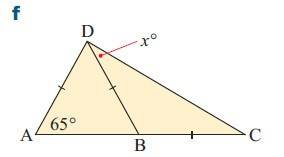# Isosceles triangle with angle bisector

## Homework Statement

Find x[/B]a+b+c=180[/B]

## The Attempt at a Solution

How do I find x in DBC?

SammyS
Staff Emeritus
Homework Helper
Gold Member

## Homework Statement

Find x[/B]
View attachment 104594

a+b+c=180[/B]

## The Attempt at a Solution

How do I find x in DBC?
What is the measure of angle CBD ?

What is the measure of angle CBD ?
What is the measure of angle CBD ?

angle bisector -> angle on a line 180 degrees. 180-65=115
x= 180-115=65, 65/2=32.5
CBD=32.5+115+32.5

x=32.5

SammyS
Staff Emeritus
Homework Helper
Gold Member
angle bisector -> angle on a line 180 degrees. 180-65=115
x= 180-115=65, 65/2=32.5
CBD=32.5+115+32.5

x=32.5
Actually, I asked about angle CBD, ( ∠ CBD ), not triangle CBD ( Δ CBD ).

Maybe, that's what you mean by
" angle bisector -> angle on a line 180 degrees. 180-65=115 ",​
but an angle bisector cuts an angle exactly into two angle of equal measure.

So, yes, .∠ CBD = 180° - 65° = 115° .

It's really very confusing, and incorrect, when you state the following.
CBD=32.5+115+32.5